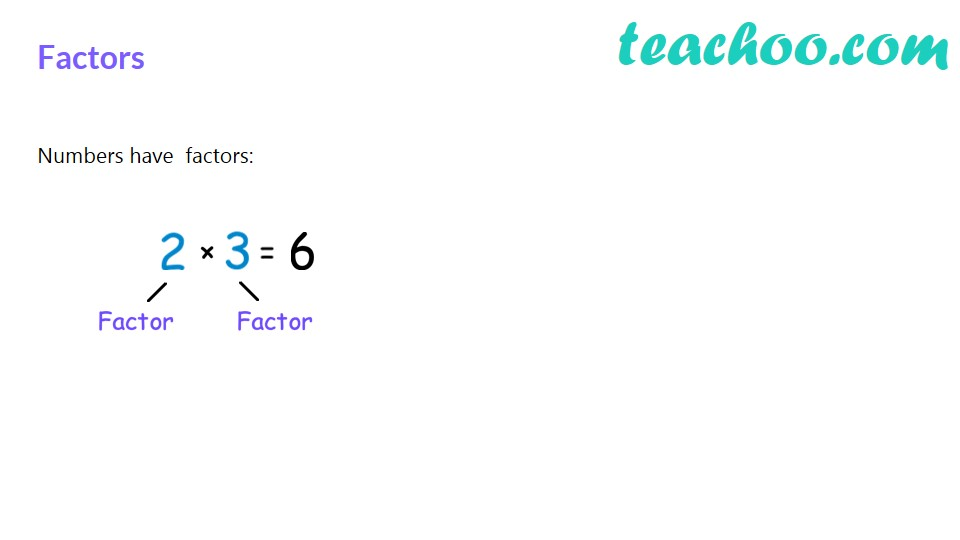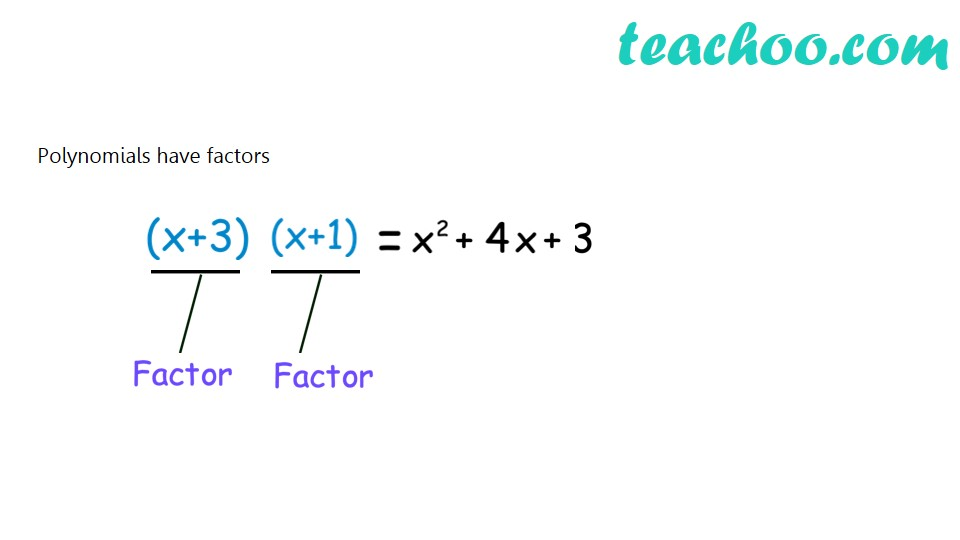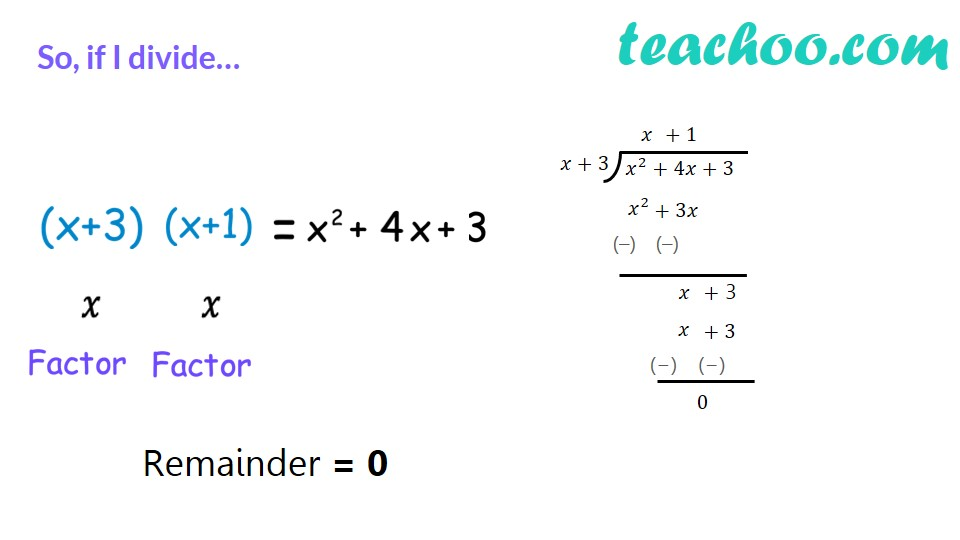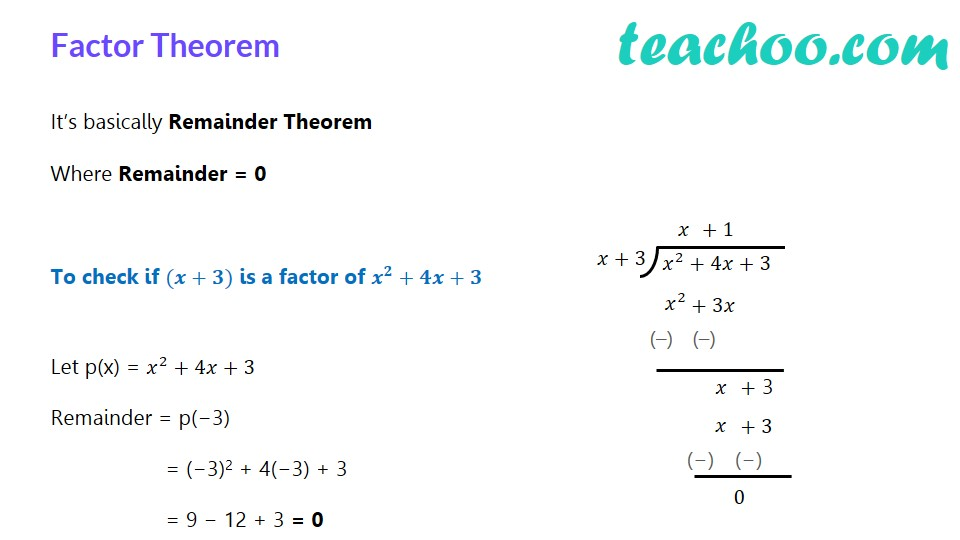Check if factor

Chapter 2 Class 9 Polynomials
Concept wiseTo check if g(x) is a factor of p(x),

If on dividing p(x) by g(x), we get remainder 0,

Then g(x) is a factor of p(x)

Let us take an example

p(x) = x 3 − 3x 2   + 4x + 10

g(x) = x + 1

Is g(x) a factor of p(x)?

We find remainder by Remainder theorem

Putting g(x) = 0

x + 1 = 0

x =  − 1

So, Remainder = p(− 1)

= (−1) 3   − 3(− 1) 2 + 4(−1) + 10

= (−1)  − 3 x 1  − 4 + 10

= −1 − 3 − 4 + 10

= 2

Since Remainder ≠ 0

It is not a factor.

Let us check more examples. click next to practice more questions.

Learn in your speed, with individual attention - Teachoo Maths 1-on-1 Class